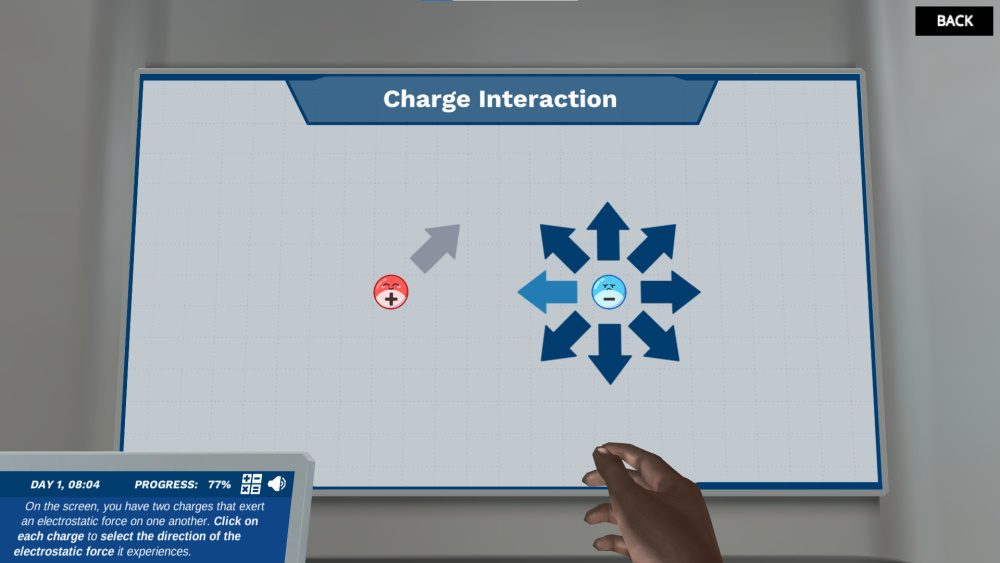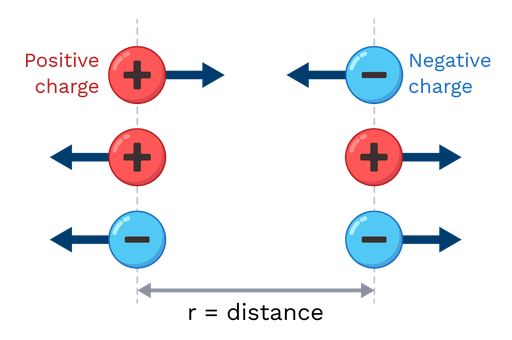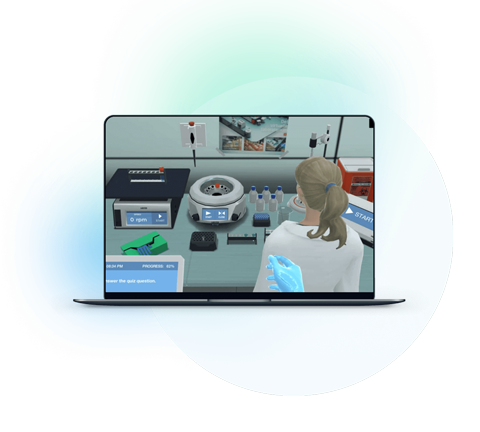Blog

# 5 Ways to Make Coulomb’s Law a More Approachable Topic for Students

Usama Attiq
Teaching with Labster
November 3, 2022

## Introduction: Coulomb’s law

Electrostatics is the branch of physics that deals with the study of electrical charges at rest. In physics, the charge is known as an electric charge. It is the property of matter that experiences a force when it is placed in an electromagnetic field. The charge has two types: positive charge and negative charge. Both types have the same number of charges but consist of opposite signs.

An atom contains a positive charge when it has more protons than electrons. In the same way, when an atom contains more electrons than protons, it has a negative charge.

An inverse square law states that a physical quantity reduces with an inverse square of the distance from the source. An example of inverse square law is Coulomb’s law.

Coulomb’s law states that the magnitude of the electrostatic force of attraction or repulsion is directly proportional to the product of the magnitudes of the charges and inversely proportional to the square of the distance between two charges.

F = k. (Q1) . (Q2) / r2

In the above equation, F is the force of attraction and repulsion between two charged bodies, (Q1) and (Q2) are the electrical charges, k is the proportionality constant, and r is the distance between the two charge bodies.

Sometimes, students may find some difficulties while learning Coulomb’s law. At Labster, we compiled all the reasons that make this topic difficult to understand. We also explain five methods to make this topic easier for students. In the end, we will convince you why a virtual lab simulation is important for students as well as for teachers to convey the topic of Coulomb’s law in the class.Figure: An image shows the charge interaction between the positive and negative charge from Labster virtual laboratory of Coulomb’s law

## Why Coulomb’s law can be tricky to learn

There are three main reasons that make Coulomb’s law a difficult topic, even for hardworking students.

### 1. It feels abstract

Many abstract concepts are used in Coulomb’s law. These are charges, electrostatic force, electrons, and protons that students cannot see with the naked eye. It is hard for students to believe in abstract concepts. During studying this topic, they cannot imagine the structure of electric charges which makes this topic difficult to learn.

### 2. It’s content-heavy

Some students may experience difficulties while learning Coulomb’s law because it’s content-heavy. The topic consists of basic law, electrostatic forces, examples, formulas, and limitations that can be hard for students to remember. So, students may lose interest in reading Coulomb’s law due to the complexity of the topic.

### 3. Solving numerical problems and graph representation

In Coulomb’s law, you have to solve different numerical problems by using the formula. Also, it is important to know about the graph representation of the electrostatic force of attraction and repulsion between the charges. Students may find it confusing and boring to solve the numerical and make a graph to represent Coulomb’s law.

## 5 ways to make Coulomb’s law a more approachable topic to understand

We are familiar with the difficulties that students experience during studying Coulomb’s law. So, there are five methods that make this topic simpler and more interesting to understand.

### 1. Talk about the people behind the science

Charles Augustin de Coulomb:

Charles Augustin de Coulomb was a French physicist who provided a mathematical explanation of the two electrically charged bodies. He was the first person who studied electrostatics. In 1785, he introduced an equation that shows the force causing two bodies to attract or repel each other. It is known as Coulomb’s inverse square law or Coulomb’s law. He represented the three reports related to electricity and described the laws of attraction and repulsion between the charges and bodies. He developed a device known as “dubbed the torsion balance”. It helped to measure the charges and estimate the force of attraction and repulsion between the charges.

### 2. Basic concept and properties of Coulomb law

Students need to learn the interesting facts and properties of Coulomb’s law. The electrostatic force is expressed through the unit Newton. Electrostatic force is a vector quantity that has both magnitude as well as direction. The direction of the electrostatic force depends upon the charge of the particles, whether they are similar or opposite. It is necessary to know that the electrostatic force is not constant. The electrostatic force is the main reason that explains the distance between the charges.

Coulomb’s law represents accurate information about the force present between the two bodies. Coulomb’s first law states that two objects with the same charges repel each other while two objects with different charges attract each other. Coulomb’s second law states that the force of attraction or repulsion between two objects is directly proportional to the magnitude between the objects and inversely proportional to the square of the distance between two objects.

There is a new constant in Coulomb’s law known as the permittivity of free space. It is also called as permittivity of the vacuum.Figure: An image explaining Coulomb’s law from Labster theory

Coulomb explains the properties of the electric force of the charge at the rest state. These are the following:

1. Two bodies with the same charges repel each other while two bodies with opposite charges attract each other. It means that two negative charges repel each other, and a positive charge attracts the negative charge.

2. The force between two charges is either attractive or repulsive.

3. The force is inversely proportional to the distance between the charges. If the distance between the charges increases, the electrostatic force between the charges becomes weaker.

4. The magnitude of the force is proportional to the magnitude of both charges. The unit Coulomb (C) is used to measure the electrostatic charge.

Limitations of Coulomb’s law: Coulomb’s law has four main limitations. Students should know the limitations of Coulomb’s law that help them to understand the topic properly.

• If the solvent molecules between the two charged objects are larger, Coulomb’s law is applicable.

• Coulomb’s law is only valid if the charged objects are at rest state.

• Another limitation of Coulomb’s law is that it is not applicable if the charges have an arbitrary shape. The reason is that we are not able to determine the distance between the two charges when they have arbitrary shapes.

• Coulomb’s law is valid if the case follows the inverse square law.

### 3. Relate to real world

Students find Coulomb’s law more interesting when they learn real examples related to the topic. They can perform these experiments in class to know that Coulomb’s law is really applicable in daily life.

1. When a glass rod is rubbed with silk cloth or woolen piece, it gets charged. The charged rod can attract or repulse other objects. The charged glass rod can attract the positive charge paper towards itself. It is due to Coulomb’s law.

2. Another example of Coulomb’s law is the comb and small pieces of paper. When a comb is rubbed against the hair, it gets charged. This charged comb can attract small pieces of paper that have opposite charges.

3. Mixing substances in the water also follow Coulomb’s law. For instance, sodium chloride (NaCl) is a polar substance that attracts water and dissolves in it. The oil is a nonpolar substance, so it does not dissolve in water. The electrostatic interaction has also existed between the solute and the solvent.

4. Aluminum plates and Styrofoam also represent Coulomb’s law. When Styrofoam is rubbed with a wool sock, it gets negatively charged. When an aluminum plate gets closer to the Styrofoam, an electric interaction of attraction is produced between them. You can see the spark touching the aluminum plate.

### 4. Seeing is believing

Students will enjoy learning Coulomb’s law when they see the color diagrams. The diverse colors make it easier to differentiate between the charges and force of interaction between the objects.

The color image used below represents the charge interaction between the objects. Students will understand better about charge interaction by seeing this virtual image. For example, the two positive charges always repel while a positive and negative charge object always attracts. If learners see a color diagram explaining this concept, it will be better for them to understand it.

### 5. Use of virtual lab simulation

Virtual lab simulation is an advanced way to teach students about tough topics like Coulomb’s law. At Labster, we provide a 3D laboratory simulation with gamification elements like storytelling and a scoring system. It enhances the interest of students in the topic and clears their concepts.

Labster Coulomb’s law simulation explains the basic law, electrostatics forces, inverse square law, data collection method, magnitude and direction of the force, and graph representation. A virtual lab simulation is effective for students to learn Coulomb’s law and for teachers to convey the topic appropriately.Check out Labster Coulomb’s law simulation here, or get in touch to find out how you can start using virtual labs with your students.What exactly are virtual labs?